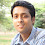### Exceptions of floating point normalization

Floating point normalization has a great usage for computing anything very near to accuracy. A floating point number is consists of:

1. Mantissa or significand.
2. Exponent.

Say, I've a number 123.75. Its a floating point number. It has integer significand, 12375 and exponent -2.

So arithmatic representation is 12375 x 10-2.

How to normalize a floating point number?

- By shifting the mantissa to left until a 1 appears in most significant bits(HO). Hence, the normalized representation will be 1.2375 x 10+2. Most of the time for normalized number this bit is hidden as it happens to be 1. This is hidden bit.

Now the question when we can't normalize a floating point number?

- There are two such situations:

1. We can't normalize zero(0). The floating point representation of Zero doesn't contain any 1 bit. However, IEEE representation for +0 and -0 has different significance.
2. We also can't normalize a floating point number whose most significant bits in mantissa are zero as well as biased exponents are also zero.
Reference: - Floating Pointgood read , however you could have explained a little more about the exceptional situation you have mentioned.we are waiting for your new post !